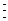# Finding the ${n}^{\text{th}}$ Term of a Sequence

The ${n}^{\text{th}}$ (or general) term of a sequence is usually denoted by the symbol ${a}_{n}$ .

Example 1:

In the sequence $2,6,18,54,...$  the first term is
${a}_{1}=2$ , the second term is ${a}_{2}=6$ and so forth.

A term is multiplied by $3$ to get the next term.

If you know the formula for the ${n}^{\text{th}}$ term of a sequence in terms of $n$ , then you can find any term.

Example 2:

${a}_{n}={n}^{2}$

First term: ${a}_{1}={1}^{2}=1$

Second term: ${a}_{2}={2}^{2}=4$Fifteenth term: ${a}_{15}={15}^{2}=225$